# HSSlive: Plus One & Plus Two Notes & Solutions for Kerala State Board

## BSEB Class 9 Maths Chapter 11 Constructions Ex 11.1 Textbook Solutions PDF: Download Bihar Board STD 9th Maths Chapter 11 Constructions Ex 11.1 Book AnswersBSEB Class 9 Maths Chapter 11 Constructions Ex 11.1 Textbook Solutions PDF: Download Bihar Board STD 9th Maths Chapter 11 Constructions Ex 11.1 Book Answers

BSEB Class 9th Maths Chapter 11 Constructions Ex 11.1 Textbooks Solutions and answers for students are now available in pdf format. Bihar Board Class 9th Maths Chapter 11 Constructions Ex 11.1 Book answers and solutions are one of the most important study materials for any student. The Bihar Board Class 9th Maths Chapter 11 Constructions Ex 11.1 books are published by the Bihar Board Publishers. These Bihar Board Class 9th Maths Chapter 11 Constructions Ex 11.1 textbooks are prepared by a group of expert faculty members. Students can download these BSEB STD 9th Maths Chapter 11 Constructions Ex 11.1 book solutions pdf online from this page.

## Bihar Board Class 9th Maths Chapter 11 Constructions Ex 11.1 Books Solutions

 Board BSEB Materials Textbook Solutions/Guide Format DOC/PDF Class 9th Subject Maths Chapter 11 Constructions Ex 11.1 Chapters All Provider Hsslive

## How to download Bihar Board Class 9th Maths Chapter 11 Constructions Ex 11.1 Textbook Solutions Answers PDF Online?

2. Click on the Bihar Board Class 9th Maths Chapter 11 Constructions Ex 11.1 Answers.
3. Look for your Bihar Board STD 9th Maths Chapter 11 Constructions Ex 11.1 Textbooks PDF.
4. Now download or read the Bihar Board Class 9th Maths Chapter 11 Constructions Ex 11.1 Textbook Solutions for PDF Free.

Find below the list of all BSEB Class 9th Maths Chapter 11 Constructions Ex 11.1 Textbook Solutions for PDF’s for you to download and prepare for the upcoming exams:

## BSEB Bihar Board Class 9th Maths Solutions Chapter 11 Constructions Ex 11.1

Question 1.
Construct an angle of 90° at the initial point of a given ray and justify the construction.
Solution:
Steps of Construction :

1. Draw a ray OA.
2. With its initial point O as centre and any radius, draw an arc CDE, cutting OA at C.3. With centre C and same radius (as in step 2), draw an arc, cutting the arc CDE at D.
4. With D as centre and the same radius, draw an arc cutting the arc CDE at E.
5. With D and E as centres, and any. convenient radius (more than 12 DE ), draw two arcs intersecting at P.
6. Join OP. Then ∠AOP = 90°.

Justification :
By construction, OC = CD = OD
∴ ∆ OCD is an equilateral triangle. So, ∠COD = 60°.
Again, OD = DE = EO
∴ ∆ ODE is also an equilateral triangle. So, ∠DOE = 60°.
Since OP bisects ∠DOE, so ∠POD = 30°.
Now, ∠AOP = ∠COD + ∠DOP = 60° + 30° = 90°.

Question 2.
Construct an angle of 45° at the initial point of a given ray and justify the construction.
Solution:
Steps of Construction

1. Draw a ray OA.
2. With O as centre and any suitable radius draw an arc cutting OA at B.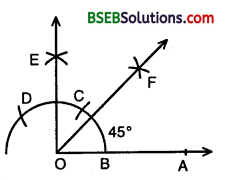3. With B as centre and same radius cut the previous drawn arc at C and then with C as centre and same radius cut the arc at D.
4. With C as centre and radius more than half CD draw an arc.
5. With D as centre and same radius draw another arc to ut the previous arc at E.
6. Join OE. Then ∠AOE = 90°.
7. Draw the bisector OF of ∠AOE. Then ∠AOF = 45°.

Justification :
By construction, ∠AOE = 90° and OF is the bisector of ∠AOE
∴ ∠AOF = 12∠AOE = 12 x 90° = 45°.

Question 3.
Construct the angles of the following measurements :
(i) 30°
(ii) 221°2
(iii) 15°.
Solution:
(i) Steps of Construction :

• Draw a ray OA.
• With its initial point O as centre and anyx radius, draw an arc, cutting OA at C.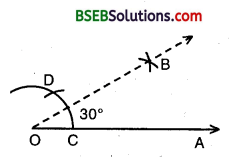• With centre C and same radius (as in step 2). Draw an arc, cutting the arc of step 2 in D.
• With C and D as centres, and any convenient radius (more than 12 CD), draw two arcs intersecting at B.
• Join OB. Then ∠AOB = 30°.

(ii) Steps of Construction :

• Draw an angle AOB = 90°.
• Draw the bisector OC of ∠AOB, then ∠AOC = 45°.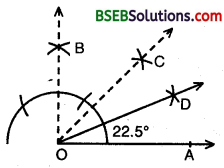• Bisect ∠AOC, such that ∠AOD = ∠COD = 22.5°.
Thus, ∠AOD = 22.5°.

(iii) Steps of Construction :

• Construct an ∠AOB = 60°.
• Bisect ∠AOB so that ∠AOC = ∠BOC = 30°.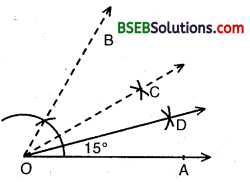• Bisect ∠AOC, so that ∠AOD = ∠COD = 15°.
Thus, ∠AOD = 15°.

Question 4.
Construct the following angles and verify by measuring them by a protractor :
(i) 75°
(ii) 105°
(iii) 135°
Solution:
(i) Steps of Construction :

• Draw a ray OA.
• Construct ∠AOB = 60°.
• Construct ∠AOP = 90°.
• Bisect ∠BOP so that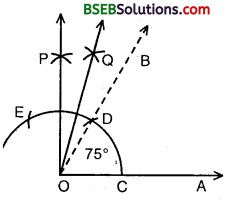• So, we obtain ∠AOQ = ∠AOP + ∠BOQ = 60° + 15° = 75°.

Verification :
On measuring ∠AOQ, with the protractor, we find ∠AOQ – 75°.

(ii) Steps of Construction :

• Draw a line segment XY.
• Construct ∠XYT = 120° and ∠XYS = 90°, so that ∠SYT = ∠XYT – ∠XYS = 120° – 90° = 30°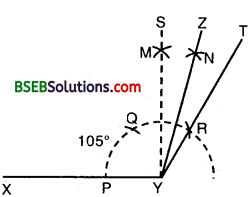• Bisect angle SYT, by drawing its bisector YZ.
• Then ∠XYZ is the required angle of 105°.

(iii) Steps of Construction :

• Draw ∠AOE = 90°. Then, ∠LOE = 90°.• Draw the bisector OF of ∠LOE.
• Then, ∠AOF = 135°.

Question 5.
Construct an equilateral triangle, given its side and justify the construction.
Solution:
Let us draw an equilateral triangle of side 4.6 cm (say).
Steps of Construction :

1. Draw BC = 4.6 cm.
2. With B and C as centres and radii equal to BC = 4.6 cm, draw two arcs on the same side of BC, intersecting each- other at A.3. Join AB and AC.
4. Then, ABC is the required equilateral triangle.

Justification :
Since by construction :
AB = BC = CA = 4.6 cm.
∴ ∆ ABC is an equilateral triangle.

## Bihar Board Class 9th Maths Chapter 11 Constructions Ex 11.1 Textbooks for Exam Preparations

Bihar Board Class 9th Maths Chapter 11 Constructions Ex 11.1 Textbook Solutions can be of great help in your Bihar Board Class 9th Maths Chapter 11 Constructions Ex 11.1 exam preparation. The BSEB STD 9th Maths Chapter 11 Constructions Ex 11.1 Textbooks study material, used with the English medium textbooks, can help you complete the entire Class 9th Maths Chapter 11 Constructions Ex 11.1 Books State Board syllabus with maximum efficiency.

## FAQs Regarding Bihar Board Class 9th Maths Chapter 11 Constructions Ex 11.1 Textbook Solutions

#### Can we get a Bihar Board Book PDF for all Classes?

Yes you can get Bihar Board Text Book PDF for all classes using the links provided in the above article.

## Important Terms

Bihar Board Class 9th Maths Chapter 11 Constructions Ex 11.1, BSEB Class 9th Maths Chapter 11 Constructions Ex 11.1 Textbooks, Bihar Board Class 9th Maths Chapter 11 Constructions Ex 11.1, Bihar Board Class 9th Maths Chapter 11 Constructions Ex 11.1 Textbook solutions, BSEB Class 9th Maths Chapter 11 Constructions Ex 11.1 Textbooks Solutions, Bihar Board STD 9th Maths Chapter 11 Constructions Ex 11.1, BSEB STD 9th Maths Chapter 11 Constructions Ex 11.1 Textbooks, Bihar Board STD 9th Maths Chapter 11 Constructions Ex 11.1, Bihar Board STD 9th Maths Chapter 11 Constructions Ex 11.1 Textbook solutions, BSEB STD 9th Maths Chapter 11 Constructions Ex 11.1 Textbooks Solutions,
Share: